# Joint Multilevel Turbo Equalization and Continuous Phase Frequency Shift Keying

## Abstract

A novel type of turbo coded modulation scheme, called multilevel turbo coded-continuous phase frequency shift keying (MLTC-CPFSK), is designed to improve the overall bit error rate (BER) and bandwidth efficiency. Then, this scheme is combined with a new double decision feedback equalizer (DDFE) to remove the interference and to enhance BER performance for the intersymbol interference (ISI) channels. The entire communication scheme is called multilevel turbo equalization-continuous phase frequency shift keying (MLTEQ-CPFSK). In these schemes, parallel input data sequences are encoded using the multilevel scheme and mapped to CPFSK signals to obtain a powerful code with phase continuity over the air. The performances of both MLTC-CPFSK and MLTEQ-CPFSK systems were simulated over nonfrequency and frequency-selective channels, respectively. The superiority of the two level turbo codes with 4CPFSK modulation is shown against the trellis-coded 4CPFSK, multilevel convolutional coded 4CPFSK, and TTCM schemes. Finally, the bit error rate curve of MLTEQ-CPFSK system over Proakis B channel is depicted and ISI cancellation performance of DDFE equalizer is shown against linear and decision feedback equalizers

## 1. Introduction

With the development of the wireless communication industry, wireless data communications have become a very important research area for many scientists. As a result, tremendous improvements have occurred in coding, modulation, and signal processing subsystems to provide burst rates along with power efficiency at low bit error rates. First, conventional turbo code was found to be very attractive in the last decade , since turbo code reached theoretical limits in an iterative fashion at low signal-to-noise ratio with a cost of a low code rate and bandwidth expansion. Several years later, the compensation for bandwidth expansion and the low code rate was realized by applying multilevel and trellis-coded modulation to turbo code, known as multilevel turbo codes (MLTCs) [2, 3] and turbo trellis-coded modulation (TTCM) [4, 5], respectively, in the literature. These techniques increase the spectral efficiency of the coding via concatenating higher-order modulation using PSK or QAM modulations ; however, these communication models have phase jumps in their modulated signals. Continuous phase modulation (CPM) has explicit advantages in deep space and satellite communications, such as having low spectral occupancy property. Thus, to improve the bandwith usage further, MLTC design is concatenated and investigated with CPM modulation in this research.

MLTC is modeled by applying separate turbo encoders at each level. Each turbo encoder processes the information sequence simultaneously. For each level of the mutilevel encoder, there exists a corresponding decoder defined as a stage. The output of one stage is utilized at the decoder of the following stage in the decoding flow, known as multistage decoding .

The CPM model that is used with the MLTC is composed of a continuous-phase encoder (CPE) and a memoryless mapper (MM). The CPE is a convolutional encoder producing codeword sequences that are mapped onto waveforms by the MM, creating a continuous-phase signal. CPE-related schemes have better BER performance than systems using the traditional approach for a given number of trellis states due to larger Euclidean distances. When the decomposed structure of CPM is considered, joint trellis coded and CPM, and joint multilevel convolutional code and CPM systems can be designed as in [8, 9].

For achieving a low bit error rate (BER) over a severe ISI channel, the double decision feedback equalization is designed for MLTC-CPFSK system . It is well know that maximum a posteriori probability (MAP) and soft output Viterbi algorithm-based equalizers are very effective, but having very high complexity, which is not applicable to our design since our transmission scheme has high complexity. Performances of traditional low-complexity equalizers such as linear equalizer (LE) and decision feedback equalizers (DFE) are not effective under severe channel conditions. To close the performance gap between high- and low-complexity equalizers, DDFE is proposed and implemented into the MLTC-CPFSK system. Thus, the effect of a severe ISI channel is mitigated by the equalization process and then the equalized information passes through the MAP algorithm-based decoders which decode the two encoded streams by exchanging the soft decisions.

In this paper, Section 2 explains the design of multilevel turbo encoder with CPFSK modulation. Section 3 describes the DDFE-based turbo equalization receiver scheme. In Section 4,the performances of the proposed MLTC-CPFSK and MLTEQ-CPFSK schemes are presented over AWGN, Rician, Rayleigh, and Proakis B channels, respectively, and the conclusion is stated at the last section.

## 2. The Design of Multilevel Turbo Encoder Using CPFSK

M-ary continuous phase frequency shift keying (M-CPFSK) is a special form of M dimensional CPM. In the literature, Rimoldi firstly derived the tilted-phase representation of CPM in , with the information-bearing phase given by(1)

The modulation index h is equal to J/P, where J and P are relatively prime integers. X is an input sequence of M-ary symbols,. T is the channel symbol period. In CPFSK design, modulation indexis considered and the frequency pulse is a rectangular pulse of duration LT and height 1/(2LT), yielding a linearly increasing/decreasing instantaneous phase. In this design, full response CPFSK modulator is considered. The phase response functionis a continuous and monotonically increasing function subject to the constraints(2)

where L is an integer that denotes the number of memory units in CPE. The phase response is usually defined in terms of the integral of a frequency pulseof duration LT, that is,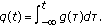For full response signaling L equals to 1, and for partial response systems L is greater than 1. Finally, the transmitted signalis derived as(3)

whereis the asymmetric carrier frequency asandis the carrier frequency.is the energy per channel symbol andis the initial carrier phase. We assume thatis an integer; this condition leads to a simplification when using the equivalent representation of the CPM waveform.

Multilevel turbo code using CPFSK modulation consists of many parallel turbo encoder/decoder levels. In Figure 1, high-level block diagram of designed transceiver is shown for n level case. There exists a binary turbo encoder at every level of the multilevel turbo encoder, and there is a continuous-phase encoder serially connected to the turbo encoder at the last level. Based on Rimoldi's model and the assumption in , CPE would be a convolutional encoder. In this model, the outside encoder is designed to maximize the Euclidean distance between output signals whereas CPE is used to shape the modulated signal's spectrum. In the CPFSK design, the state of CPE is binary and it changes with time. The phase of the modulated signal depends on the memoryless modulator. The first process of the transmitter is that the information sequence is converted from serial to parallel in the multilevel scheme. Then, each turbo encoder processes the information sequence simultaneously. Additionally, the outputs of the last turbo encoders are run through the CPE. The outputs of all encoders' outputs and CPE output are mapped to CPFSK signals. In Figure 4, the two-level multilevel turbo transmission system (2LTC-4CPFSK) is illustrated in detail since this research simulations were performed on a two-level model. In each level, a 1/3 turbo encoder is demonstrated with recursive systematic convolutional (RSC) encoders having memory sizeas in Figure 4(a). For mapping the encoders' outputs to 4CPFSK signals, the first and second bits are taken from the first and second level of turbo encoder output, respectively. The third bit is obtained from the output of the CPE. Thus, based on the output of the encoders,, and, the CPFSK modulated signalsare transmitted at four different frequenciesand. The initial and ending physical tilted phases areandas shown in Figure 5. At the receiver, 4CPFSK signal constellation partitioning is optimized to provide low BER for AWGN and fading channels as in . The signal set partitioning technique for 2LTC-4CPFSK signals is as follows: depending on the estimated output bit of the first-level turbo decoder is whetheror,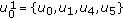orsignal set is chosen, respectively. Then, depending on the estimated second-level turbo encoder output bitand the CPE output bit, the transmitted signal is determined as shown in Figure 5.

## 3. Turbo Equalization Receiver Scheme

For the k th symbol interval, a set of basis functions was used to find the coordinates in signal space and to form the vectorin each signaling interval. Let the transmitted MLTC-CPFSK symbol sequence beand the corresponding received sequence be, where the k th received vector element equals to. In this case, the channel output during the k th symbol interval can be expressed as(4)

whereis k th noise vector element of the noise sequenceand its elements are additive white Gaussian noise with anpower spectral density, Es is the signal energy per symbol,is Rician fading amplitude, which varies by Rician probability distribution function as in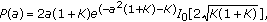(5)

where K is the Rician factor in terms of dB. We assume that the demodulator operates over one symbol interval, which yields a discrete memoryless channel. At the receiver, the corrupted MLTC-CPFSK signals are processed by the demodulator and MAP decoder to extract the information sequence.

MLTEQ-CPFSK scheme is applied to Proakis B channel. This channel is time-invariant ISI channel havingcasual,anticasual terms and is known as severe ISI channel with 3 main taps and no precursor and postcursor taps . The output of the channel is equal to(6)

whereare the coefficients of the equivalent discrete channel.

After the M-CPFSK modulated signals are run through the channel, they are demodulated and then noisy demodulator outputs are evaluated for every equalization and decoding process. The following is the high-level summary of the equalization and decoding process. In the first step, the probabilities of received signal being zero and one is computed as in (7) and then, the probabilities are mapped torange via (8). In the second step, the computation of the equivalent discrete channel taps is explained when the channel conditions are known for the traditional decision feedback equalizer. In our application and real applications, the channel information is not known. Thus, the estimation process of the channel via LMS algorithm is performed and described. The coefficient vectors of the filters are defined from (14) and their adaptation is explained from (15). The DDFE equalization output is derived with (17). Finally, the equalized information is processed by the MAP decoder as in (18).

At the receiver, the probabilities of the corrupted received signals being zero and one are computed as follows: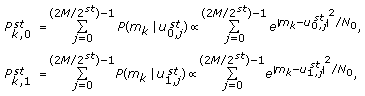(7)

whereandindicate zero and one probabilities of the received signal at time k and stage st. The partitioning stage is equal to.,are the selected signal sets at stage st.

In multilevel coding scheme, each digit of binary correspondence of CPFSK signals matches to one stage from the most significant to the least significant while the stage number increases. Signal set is partitioned into the subsets due to each binary digit matching stage depending on whether it is 0 or 1. After computing the one and zero probabilities, received signals are mapped to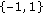range and then sent to the equalization and decoding process at each level,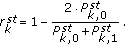(8)

As shown in Figure 2, DDFE structure mainly consists of 3 linear transversal filters: the feed forward (FF) filter, and two feedback filters (FB), a channel interleaver (), deinterleaver (), and two delay components. The decoder structure is made of two interleavers (), two deinterleavers (), a demultiplexer, and two soft input soft output (SISO) decoders which exchange priori information as indicated in more detail in Figure 3.

In order to reduce the notation of the equations and figures, the notation is not changed when the information is processed by the interleavers. Only the channel output feeds the equalizer at the first iteration, therefore, the equalizer uses training sequence to operate for the initial process. For further iterations, the FF filter is fed by the channel output and the channel estimator output. The channel estimator uses both the hard decision of the first decoder and the channel output to estimate the channel information. The first FB filter uses the hard decision of the first decoderwhereas the second FB filter uses the hard decision of the second decoder.

In order to perform the conventional DFE equalization, error propagation has to be ignored, which means. The coefficient of the equalizer is computed in  by using mean square error (MSE), which is based on the minimization of the difference between the equalized data () and the hard decision of the first decoderas follows:(9)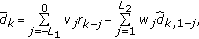(10)

whereandare the numbers of feedforward and feedback coefficients, respectively. v is the coefficient of the FF filter, where w is the coefficient of the FB filter. By using first orthogonality principle, the feedforward coefficients of the filter are computed. This principle yields to the following set of linear equations: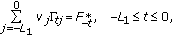(11)

where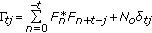(12)

whereandis the channel tap. The coefficient of the FB filter is computed by the second orthogonality principle,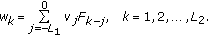(13)

Since the equivalent discrete channel taps are unknown in most of the communication applications, the filter coefficient cannot be computed from the equation above.

We selected LMS algorithm to determine the filter coefficients because of its less complexity and high accuracy on time-invariant channels at large frame sizes. By LMS algorithm, the coefficients of channel and feedback filters are estimated from the corrupted transmitted signal and the hard decisions of the decoders after certain latency () is introduced to the system. After the first iteration, the coefficient vectors of the FF, first FB, and second FBfilters are computed, respectively, as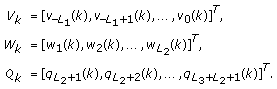(14)

In LMS algorithm, the coefficients of the FF and FB filters are adapted as follows:(15)

whereis the step size of the LMS algorithm, and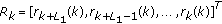is the vector of the transmitted signal, and the vectors below are the hard-decision vectors of the decoders from the previous iteration,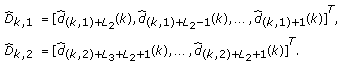(16)

After the corrupted transmitted signals are filtered by FF and first FB filters as shown in (10), it is deinterleaved ()and subtracted from the output of the second FB filter to obtain DDFE outputas below,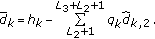(17)

During the initializing period, the coefficients of the FF filter at the first iteration are estimated from the training sequence by the LMS criterion due to the fact that the hard decision of the decoder does not exist at the first iteration. Therefore, the DDFE structure behaves as a linear equalizer fed by the training sequence at the first iteration.

Eventually, the equalized information sequencesare passed through the SISO decoders.In SISO decoders, the MAP algorithm calculates the a posteriori probability of each bit at each decoding process . At the last iteration, hard decision is computed by using the second decoder outputas follows:(18)

## 4. Simulation Results

The performance of the two-level turbo coded 4CPFSK system is shown by plotting the bit error rate versus signal-to-noise ratio in Figure 6. Joint two-level turbo code and 4CPFSK scheme with random interleaver size N = 1024, generator sequence (37, 21) in octal form, and overall rate 2/3 was simulated for AWGN, Rician (Rician fading parameter K = 10 dB) and Rayleigh channels. Then, the proposed 2LTC-4CPFSK scheme was compared to the known existing multilevel schemes using CPFSK showed in [8, 9], called ref-1 and ref-2, respectively. The code on reference one (ref-1) is binary trellis-coded 4-ary CPFSK scheme with overall rate 2/3, and the code labeled (S-5) in reference two (ref-2) is the combined multilevel convolutional code and 4CPFSK. The proposed system has much better performance than ref-1 and satisfactory coding gain over ref-2 for all channel conditions as stated in Table 1. The comparison among these systems was revealed for the fixed bit error rate. For instance, the coding gain under AWGN channel between MLTC-CPFSK and ref-1 is 3.3, 4.05, and 4.3 dB for iteration one to three, respectively.Table 1 Coding gains (in dB) over reference systems for .

The proposed 2LTC-CPFSK method was also compared with the bandwidth efficient TTCM scheme, which is shown in Figure 7. The TTCM model in  was simulated with 8 states, N = 1024, 2048 bits for overall rate 1 to provide the BER performance for AWGN and Rician (K = 10 dB) channels. To have a better comparison with TTCM model at the same overall rate, we have performed MLTC-CPFSK simulation with the same parameters as given above, except puncturing that used this time to achieve overall rate 1. The comparison indicates that MLTC-CPFSK system has 0.9, 1.2, and 1.3 dB coding gain against TTCM system for first, second, and third iterations, respectively, over AWGN channel. Also, our design has 1.7–2 dB gain over Rician channel. This important performance difference is achieved by MLTC-CPFSK system due to the fact that the concatenation of the powerful multilevel turbo codes and the CPE encoder yields higher Hamming distance and leads to good error performance for both AWGN and Rician channels.

The BER performance of 2LTEQ-4CPFSK is depicted over Proakis B channel for interleaver size N = 2048 frame size in Figure 8. Aggressive performance of the designed MLTEQ-CPFSK model was generated under severe ISI channel such as BERwas achieved at SNR 10.5 dB at the sixth iteration. It is illustrated that significant amount of gain is achieved at each iteration by reducing the frequency dispersive effects of Proakis B channel. DDFE equalizer has 0.8 dB and 2.1 dB gains at BERover conventional DFE and minimum mean square sequence error-based LE equalizers, respectively. DDFE equalizer provides gain at a cost of introducing additional feedback filter and delay into the system when compared to the complexity of the DFE equalizer. The overall delay of the proposed systems will be minimized to be employed in some real applications; however, the proposed systems are suitable for mobile data communications, video, and audio broadcasting.

## 5. Conclusion

We have presented multilevel turbo codes scheme with CPFSK modulation, and joint multilevel turbo equalization scheme with CPFSK modulation in this paper. MLTC-CPFSK design compensates the requirement of large frame size and high iteration number to obtain low BER at low SNR for turbo codes by adding complexity and slight latency due to the multistage structure. As shown in Figure 7, MLTC-CPFSK model achievesBER performance at the third iteration when SNR equals to 3 dB and 3.7 dB for AWGN and Rician channels, respectively. When we compared our model with the well-known multilevel coded CPFSK and TTCM schemes in the literature, we observed important coding gains with the simulation results. Furthermore, low-complexity DDFE equalizer was designed and its good interference cancellation performance was presented against LE and DFE equalizers. Eventually, satisfactory performance results for MLTEQ-CPFSK scheme is demonstrated for severe ISI channels.

## References

1. Berrou C, Glavieux A, Thitimajshima P: Near Shannon limit error-correcting coding and decoding: turbo-codes (1). Proceedings of IEEE International Conference on Communications (ICC '93), May 1993, Geneva, Switzerland 1064-1070.

2. Papke L, Fazel K: Combined multilevel turbo-code with MR-modulation. Proceedings of IEEE International Conference on Communication (ICC '95), June 1995, Seattle, Wash, USA 2: 668-672.

3. Divsalar D, Pollara F: Multiple turbo codes. Proceedings of IEEE Military Communications Conference (MILCOM '95), November 1995, San Diego, Calif, USA 1: 279-285.

4. Robertson P, Wörz T: Bandwidth-efficient turbo trellis-coded modulation using punctured component codes. IEEE Journal on Selected Areas in Communications 1998, 16(2):206-218. 10.1109/49.661109

5. Blackert WJ, Wilson SG: Turbo trellis coded modulation. Proceedings of the Conference on Information Signals and System (CISS '96), March 1996, Princeton, NJ, USA

6. Wachsmann U, Fischer RFH, Huber JB: Multilevel codes: theoretical concepts and practical design rules. IEEE Transactions on Information Theory 1999, 45(5):1361-1391. 10.1109/18.771140

7. Bayat O, Shafai B, Ucan ON: Iterative equalization of frequency selective channels. Proceedings of IEEE/Sarnoff Symposium on Advances in Wired and Wireless Communication, April 2005, Princeton, NJ, USA 33-36.

8. Naraghi-Pour M: Trellis codes for 4-ary continuous phase frequency shift keying. IEEE Transactions on Communications 1993, 41(11):1582-1587. 10.1109/26.241735

9. Altunbas I, Aygolu U: Multilevel coded CPFSK systems for AWGN and fading channels. IEEE Transactions on Communications 2000, 48(5):764-773. 10.1109/26.843189

10. Bayat O, Shafai B, Ucan ON: Reduced state equalization of multilevel turbo coded signals. Proceedings of IEEE International Conference on Acoustics, Speech and Signal Processing (ICASSP '05), March 2005, Philadelphia, Pa, USA 3: 705-708.

11. Osman O, Ucan ON, Odabasioglu N: Performance of multilevel turbo codes with group partitioning over satellite channels. IEE Proceedings: Communications 2005, 152(6):1055-1059. 10.1049/ip-com:20059063

12. Odabasioglu N, Ucan ON: Multilevel turbo coded-continuous phase frequency shift keying (MLTC–CPFSK). Computers & Electrical Engineering. In press

13. Rimoldi BE: A decomposition approach to CPM. IEEE Transactions on Information Theory 1988, 34(2):260-270. 10.1109/18.2634

14. Proakis JG: Digital Communications. 4th edition. McGraw-Hill, New York, NY, USA; 2000.

15. Bayat O, Hisham A, Ucan ON, Osman O: Performance of turbo coded signals over fading channels. Journal of Electrical & Electronics Engineering 2002, 2(1):417-422.

## Author information

Authors

### Corresponding author

Correspondence to Oguz Bayat.

## Rights and permissions

Reprints and Permissions

Bayat, O., Odabasioglu, N., Osman, O. et al. Joint Multilevel Turbo Equalization and Continuous Phase Frequency Shift Keying. J Wireless Com Network 2008, 458785 (2009). https://doi.org/10.1155/2008/458785

• Revised:

• Accepted:

• Published:

• DOI: https://doi.org/10.1155/2008/458785

### Keywords

• Turbo Code
• Continuous Phase Modulation
• Decision Feedback Equalizer
• Turbo Encoder
• Feed Forward